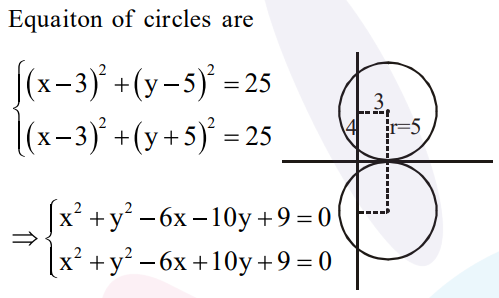# A circle touching the`
Question:

A circle touching the $\mathrm{x}$-axis at $(3,0)$ and making an intercept of length 8 on the $y$-axis passes through the point:

1. (3,10)

2. (2,3)

3. $(1,5)$

4. $(3,5)$

Correct Option: 1,

Solution: